### Home > CALC > Chapter 9 > Lesson 9.2.1 > Problem9-56

9-56.
1. Examine the integrals below. Consider the multiple tools available for integrating and use the best strategy. After evaluating each integral, write a short description of your method. Homework Help ✎

1.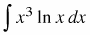2.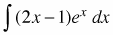3.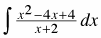4.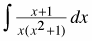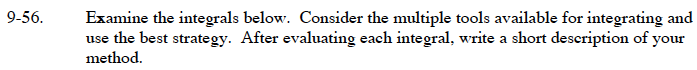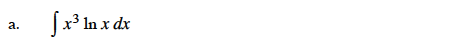Use integration by parts.

Let f = ln(x) and dg = x3dx.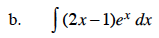Let f = 2x − 1 and dg = exdx.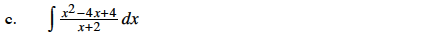Use long division to rewrite the integrand.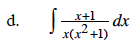Use partial fraction decomposition to rewrite the integrand in the form:

$\frac{a}{x}+\frac{bx+c}{x^2+1}$

$=\frac{1}{x}+\frac{-x+1}{x^2+1}=\frac{1}{x}-\frac{x}{x^2+1}+\frac{1}{x^2+1}$